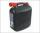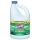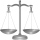# The pool

The pool contains 220 m3 of water. The pool can be emptied either:
a) 10 hours of pipe B and 8 hours of pipe A, or
b) 10 hours of pipe A and 7 hours of pipe B.

How many cubic meters of water will flow in 1 hour from pipe A and how many from pipe B?

Result

A =  15
B =  10

#### Solution:

10*B + 8*A = 220
10*A + 7*B = 220

8A+10B = 220
10A+7B = 220

A = 15
B = 10

Calculated by our linear equations calculator.

Leave us a comment of example and its solution (i.e. if it is still somewhat unclear...):Be the first to comment!#### To solve this verbal math problem are needed these knowledge from mathematics:

Looking for help with calculating harmonic mean? Looking for a statistical calculator? Do you have a system of equations and looking for calculator system of linear equations? Tip: Our volume units converter will help you with converion of volume units.

## Next similar examples:

1. BottlesThe must is sold in 5-liter and 2-liter bottles. Mr Kucera bought a total of 216 liters in 60 bottles. How many liters did Mr. Kucera buy in five-liter bottles?
2. Gasoline canisters35 liters of gasoline is to be divided into 4 canisters so that in the third canister will have 5 liters less than in the first canister, the fourth canister 10 liters more than the third canister and the second canister half of that in the first canist
3. Three workshopsThere are 2743 people working in three workshops. In the second workshop works 140 people more than in the first and in third works 4.2 times more than the second one. How many people work in each workshop?
4. Gasoline35 l of gasoline is to be divided into four canisters so that in the third canister was 5 l less than the first canister, the fourth canister 10 l more than in the third canister and the second canister half what in the first canister. How many liters of.
5. A candleA candle shop sells scented candles for \$16 each and unscented candles for \$10 each. The shop sells 28 candles today and makes \$400. a. Write a system of linear equations that represents the situation. b. Solve the system to answer the questions: How m
6. ChildrenThe group has 42 children. There are 4 more boys than girls. How many boys and girls are in the group?
7. TicketsTickets to the zoo cost \$4 for children, \$5 for teenagers and \$6 for adults. In the high season, 1200 people come to the zoo every day. On a certain day, the total revenue at the zoo was \$5300. For every 3 teenagers, 8 children went to the zoo. How many te
8. LegsCancer has 5 pairs of legs. The insect has 6 legs. 60 animals have a total of 500 legs. How much more are cancers than insects?
9. Elimination methodSolve system of linear equations by elimination method: 5/2x + 3/5y= 4/15 1/2x + 2/5y= 2/15
10. Theorem proveWe want to prove the sentence: If the natural number n is divisible by six, then n is divisible by three. From what assumption we started?
11. Linsys2Solve two equations with two unknowns: 400x+120y=147.2 350x+200y=144
12. Three unknownsSolve the system of linear equations with three unknowns: A + B + C = 14 B - A - C = 4 2A - B + C = 0
13. Home cleaningMr. Smith is cleaning up a big mess at home. In the closet, he finds a solution that is 5% bleach, and another stronger solution that is 20% bleach. For this particular job, he needs 600mL of 15% bleach. How much of each type (to the nearest mL) should he.
14. Alcohol mixingHow much 55% alcohol we must pour into 1500 g 80% alcohol to form a 60% alcohol? How much 60% alcohol created?
15. FamilyFamily has 4 children. Ondra is 3 years older than Matthew and Karlos 5 years older than the youngest Jane. We know that they are together 30 years and 3 years ago they were together 19 years. Determine how old the children are.
16. Trio weightAdelka, Barunka, and Cecilka are weight in pairs. Adelka with Barunka weighs 98 kg, Barunka with Cecilka 90 kg and Adelka with Cecilka 92 kg. How much does each of them weigh?
17. SavingsPaul has a by half greater savings than half Stanley, but the same savings as Radek. Staney save 120 CZK less than Radek. What savings have 3 boys together?